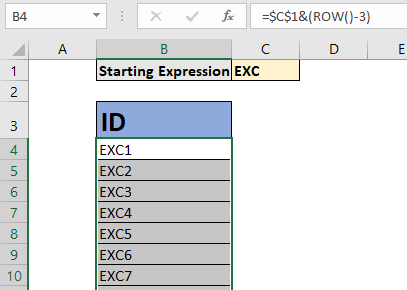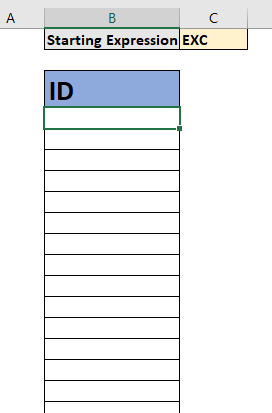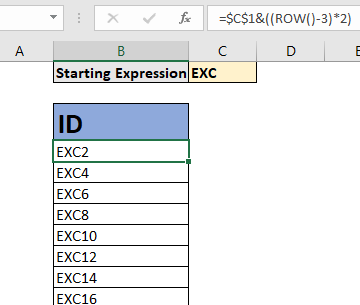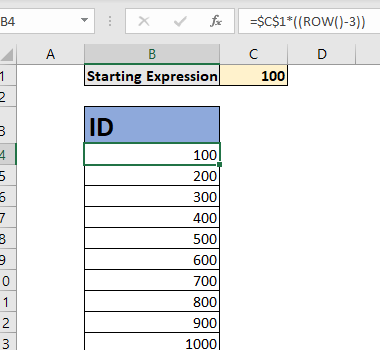# How to Increment A Value By Row or Column in ExcelIf you want to increment a value or a calculation in Excel with rows and columns as they are copied in other cells, you will need to use the ROW function (not if you have a SEQUENCE function). In this article, we will learn how you can increment any calculations with respect to row or column.

Generic Formula

 =Expression + ((ROW()-number of rows above first formula )*[steps])

Expression: This is the value, reference of expression with which you want to increment. It can be a hardcoded value or any expression that returns a valid output. It should be an absolute expression (in most cases).

Number of rows above the first formula: If you are writing this first formula in B3 then the number of rows above this formula will be 2.

[steps]: This is optional. This is the number of steps you want to jump in the next increment.

The Arithmetic operator between expression and formula can be replaced with other operators to suit the requirements of increment.

So that we are familiar with the generic formula, let's see some examples.

## Example 1: Create an Auto Increment Formula for ID Creation.Here, I have to create an auto increment ID formula. I want to write one formula that creates ID as EXC1, EXC2, EXC3 and so on. Since we only need to start the increment by 1 and concatenate it to EXC we don't use any steps. EXC is written in Cell C1, so we will use C1 as the starting expression.

Using the general formula we write the below formula in Cell B4 and copy it down.

 =\$C\$1&(ROW()-3)

We have replaced the + operator with ampersand operator (&) since we wanted to concatenate. And since we are writing the first formula in Cell B4, we subtract 3 from ROW (). The result is here.You can see that an auto increment ID has been created. Excel has concatenated 1 to EXC and Added 1 to previous value. You will not need to write any new formula for creating an ID. You just need to copy them down.

Example 2: Increment the ID every 2 steps

If you want to increment the ID every 2 steps then you will need to write this formula.

 =\$C\$1&((ROW()-3)*2)

The result is:Example 3: Add Original Value with Every Increment

In this increment the starting expression is added to every incremented value. Here's how it will be if we want to increment the starting value of 100 as 100, 200, 300, and so on.

 =\$C\$1*(ROW()-3)

C1 contains 100.How does it work?

The technique is simple. As we know, the ROW function returns the current row number it is written in, if now parameter is supplied.  The formula above ROW() returns 4.

Next we subtract 3 from it (since there are 3 rows above the 4th row). It gives us 1. This is important. It should be a hard coded value so that it does not change as we copy the formula below.

Finally the value 1 is multiplied (or any other operation) by the starting expression. As we copy the formula below. ROW() returns 5 but subtracting value stays the same (3) and we get 2. And it continues to be the cell you want.

To add steps, we use simple multiplication.

## Increment Values By Column

In the above examples we increment by rows. It will not work if you copy them in the next column of the same row.

In the above formula we used the ROW function. Similarly, we can use the COLUMN function.

Generic Formula to Increment by Columns

 =Expression + ((COLUMN()-number of columns on left of first formula )*[steps])

Number of columns on the left of the first formula: If you are writing this first formula in B3 then the number of columns on the left of this formula will be 1.

I am not giving any examples as it will be the same as the above examples.

Alternative with SEQUENCE Function

It is a new function only available for EXCEL 365 and 2019 users. It returns an array of sequential numbers. We can use it to increment values sequentially, by rows, columns or both. And yes, you can also include the steps. Using this function you will not need to copy down the formula, as Excel 365 has auto spill functionality.

So, if you want to do the same thing as you did in Example no 3. The SEQUENCE function alternative will be:

 =\$C\$1*SEQUENCE(100)

It will automatically create 100 increment values in one go without you copying the formula. You can read about the SEQUENCE function here.So yeah, this is how you can do auto increment in Excel. You can increment the previous cell by adding 1 to it easily now or you add steps to it. I hope this article helped you. If it didn't solve your problem, let me know the difficulty you are facing, in the comments section below. I will be happy to help you. Till then keep Excelling.

Related Articles:

How To Get Sequential Row Number in Excel | Sometimes we need to get a sequential row number of in table, it can be for a serial number or anything else. In this article, we will learn how to number rows in excel from the start of data.

The SEQUENCE Function in Excel | The SEQUENCE function of Excel returns a sequence of numeric values. You can also adjust the steps in the sequence along with the rows and columns.

Increment a number in a text string in excel | If you have a large list of items and you need to increase the last number of the text of the old text in excel, you will need help of two functions TEXT and RIGHT.

Highlight Row with Bottom 3 Values with a Criteria | If you want to highlight row values with bottom 3 values and with criteria then this formula will help you out.

Popular Articles:

50 Excel Shortcuts to Increase Your Productivity | Get faster at your task. These 50 shortcuts will make you work even faster on Excel.

How to use Excel VLOOKUP Function| This is one of the most used and popular functions of excel that is used to lookup value from different ranges and sheets.

How to use the Excel COUNTIF Function| Count values with conditions using this amazing function. You don't need to filter your data to count specific values. Countif function is essential to prepare your dashboard.

How to Use SUMIF Function in Excel | This is another dashboard essential function. This helps you sum up values on specific conditions.

Terms and Conditions of use

The applications/code on this site are distributed as is and without warranties or liability. In no event shall the owner of the copyrights, or the authors of the applications/code be liable for any loss of profit, any problems or any damage resulting from the use or evaluation of the applications/code.